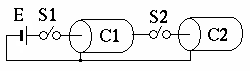# Puzzle of disappearing energy

Think about space that only has power source E1, switch S1 and S2, and two cables C1 and C2 that have same capacitance C.```1) When you close only S1 and charge power source up to voltage E,
electric charge that is stored in cable C1 is Q = C*E.
2) Open switch S1.
3) When you close switch S2, half of the electric charge of cable C1 is
going to move to cable C2 and the voltage of C1 and C2 is going to be
equal.
```
Now let's think about the energy that is stored in the cables in the circumstances 2) and 4). First of all, in the circumstance 2) the energy W1 that is stored in cable C1 is
```W1 = (1/2)*C*E*E = (1/2)*Q*Q/C
```
And next, in the circumstance 4) the total amount of energy W2 that is stored in cable C1 and C2 is
```W2 = 2*((1/2)*(Q/2)*(Q/2)/C) = (1/4)*Q*Q/C = W1/2
```
Surprisingly as soon as switch S2 is closed, the total sum of the energy in this space becames half! An opposite example of energy conservative law, which is a fundamental theory of physics, can be created easily by a simple tool like this after all. 1 meter of cable is enough to receive Nobel Prize.

However, is energy conservative law really wrong? Or electric charge conservative law that was assumed in the circumstance 4) is wrong and material itself will be disappeared?